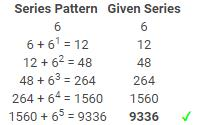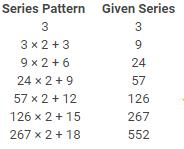Courses

# Test: Number Series - 1

## 10 Questions MCQ Test Integrated Reasoning for GMAT | Test: Number Series - 1

Description
This mock test of Test: Number Series - 1 for LR helps you for every LR entrance exam. This contains 10 Multiple Choice Questions for LR Test: Number Series - 1 (mcq) to study with solutions a complete question bank. The solved questions answers in this Test: Number Series - 1 quiz give you a good mix of easy questions and tough questions. LR students definitely take this Test: Number Series - 1 exercise for a better result in the exam. You can find other Test: Number Series - 1 extra questions, long questions & short questions for LR on EduRev as well by searching above.
QUESTION: 1

### Look at this series:4 6 12 30 90  ? 1260 What number should come?

Solution:

Logic is 4 × 1.5 = 6, 6 × 2 = 12, 12 × 2.5 = 30, 30 × 3 = 90, 90 × 3.5 = 315, 315 × 4 = 1260.

QUESTION: 2

### Look at this series: 3, 4, 7, 8, 11, 12, ... What number should come next?

Solution:

This alternating addition series begins with 3; then 1 is added to give 4; then 3 is added to give 7; then 1 is added, and so on.

QUESTION: 3

### Look at this series: 4, 11, 19, 41, ?, 161... What number should come next?

Solution:

Pattern:
4 × 2 + 3 = 11
11 × 2 – 3 = 19
19 × 2 + 3 = 41
41 × 2 – 3 = 79
79 × 2 + 3 = 161

QUESTION: 4

Look at this series: 7, 10, 8, 11, 9, 12, ... What number should come next?

Solution:

This is a simple alternating addition and subtraction series. In the first pattern, 3 is added; in the second, 2 is subtracted.

QUESTION: 5

Look at this series: 6, 12, 48, 264,1560. What number should come next?

Solution:QUESTION: 6

Look at this series: 8, 22, 8, 28, 8, ... What number should come next?

Solution:

This is a simple addition series with a random number, 8, interpolated as every other number. In the series, 6 is added to each number except 8, to arrive at the next number.

QUESTION: 7

Look at this series: 53, 53, 40, 40, 27, 27, ... What number should come next?

Solution:

A number is repeated, then it is reduced by 13 and the result is repeated.

The next number is 27-13=14.

QUESTION: 8

3  9  24  57  ? 267  552

Solution:QUESTION: 9

Look at this series: 2, 1, (1/2), (1/4), ... What number should come next?

Solution:

This is a simple division series; each number is one-half of the previous number.

In other terms to say, the number is divided by 2 successively to get the next result.

4/2 = 2
2/2 = 1
1/2 = 1/2
(1/2)/2 = 1/4
(1/4)/2 = 1/8 and so on.

QUESTION: 10

Look at this series: 1, 3, 6, 10, 15..? What number should come next?

Solution:

3 - 1 = 2,

6 - 3 = 3,

10 - 6 = 4,

15 - 10 = 5

21 - 15 = 6